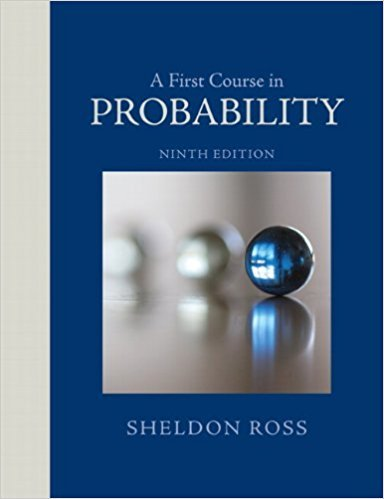×
Log in to StudySoup
Get Full Access to A First Course In Probability - 9 Edition - Chapter 4 - Problem 1te
Join StudySoup for FREE
Get Full Access to A First Course In Probability - 9 Edition - Chapter 4 - Problem 1te

Already have an account? Login here
×
Reset your password

# There are N distinct types of coupons, and each time oneISBN: 9780321794772 63

## Solution for problem 1TE Chapter 4

A First Course in Probability | 9th Edition

• Textbook Solutions
• 2901 Step-by-step solutions solved by professors and subject experts
• Get 24/7 help from StudySoup virtual teaching assistantsA First Course in Probability | 9th Edition

4 5 1 342 Reviews
28
3
Problem 1TE

Problem 1TE

There are N distinct types of coupons, and each time one is obtained it will, independently of past choices, be of type i with probability Pi, i = 1,.... N. Let T denote the number one need select to obtain at least one of each type. Compute P{T = n}.

Step-by-Step Solution:

Answer

Step 1 of 1

(a)

There aredistinct types of coupons, and each time one is obtained it will, independently of past choices, be of typewith probabilityLetdenote the number one need select to obtain at least one of each type.

We are asked to computeLetWe know the following relationship of addition rule forselections.Hence we can write that……..(1)……..(2)

Hence we can write and you can substitute the value of equation (1) and (2)Step 2 of 1

##### ISBN: 9780321794772

Since the solution to 1TE from 4 chapter was answered, more than 337 students have viewed the full step-by-step answer. This textbook survival guide was created for the textbook: A First Course in Probability , edition: 9. This full solution covers the following key subjects: type, need, coupons, denote, distinct. This expansive textbook survival guide covers 4 chapters, and 469 solutions. A First Course in Probability was written by and is associated to the ISBN: 9780321794772. The answer to “There are N distinct types of coupons, and each time one is obtained it will, independently of past choices, be of type i with probability Pi, i = 1,.... N. Let T denote the number one need select to obtain at least one of each type. Compute P{T = n}.” is broken down into a number of easy to follow steps, and 50 words. The full step-by-step solution to problem: 1TE from chapter: 4 was answered by , our top Statistics solution expert on 08/11/17, 08:57AM.

Unlock Textbook Solution

Enter your email below to unlock your verified solution to:

There are N distinct types of coupons, and each time one